# Lists in Lisp and Scheme a Lists in

• Slides: 33
Download presentationLists in Lisp and Scheme aLists in Lisp and Scheme • Lists are Lisp’s fundamental data structures, but there are others – Arrays, characters, strings, etc. – Common Lisp has moved on from being merely a LISt Processor • However, to understand Lisp and Scheme you must understand lists – common functions on them – how to build other useful data structures with them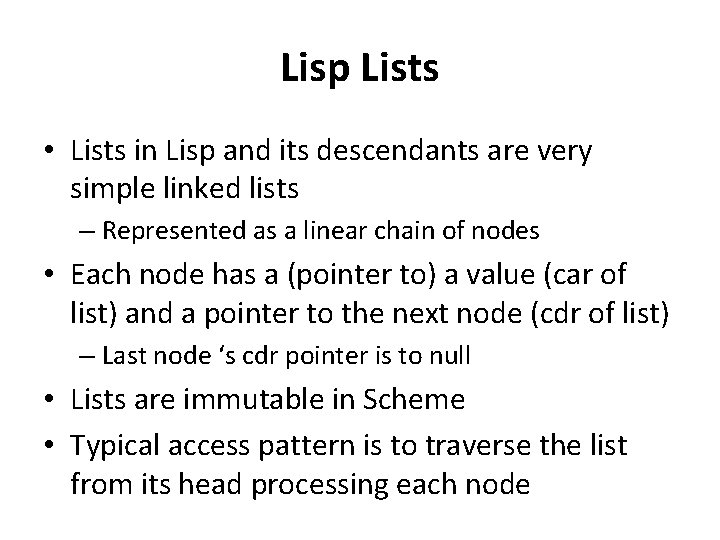Lisp Lists • Lists in Lisp and its descendants are very simple linked lists – Represented as a linear chain of nodes • Each node has a (pointer to) a value (car of list) and a pointer to the next node (cdr of list) – Last node ‘s cdr pointer is to null • Lists are immutable in Scheme • Typical access pattern is to traverse the list from its head processing each node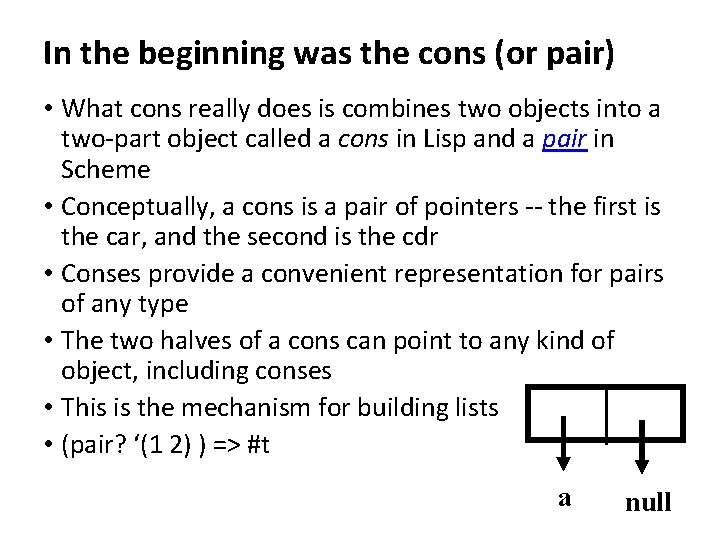In the beginning was the cons (or pair) • What cons really does is combines two objects into a two-part object called a cons in Lisp and a pair in Scheme • Conceptually, a cons is a pair of pointers -- the first is the car, and the second is the cdr • Conses provide a convenient representation for pairs of any type • The two halves of a cons can point to any kind of object, including conses • This is the mechanism for building lists • (pair? ‘(1 2) ) => #t a null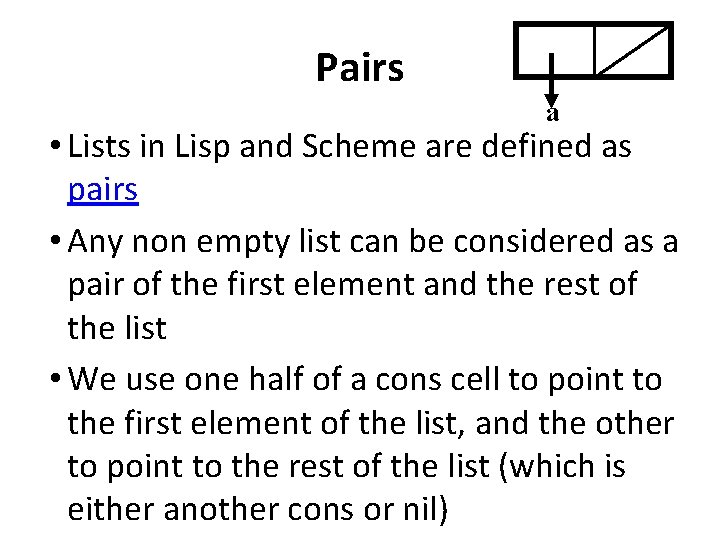Pairs a • Lists in Lisp and Scheme are defined as pairs • Any non empty list can be considered as a pair of the first element and the rest of the list • We use one half of a cons cell to point to the first element of the list, and the other to point to the rest of the list (which is either another cons or nil)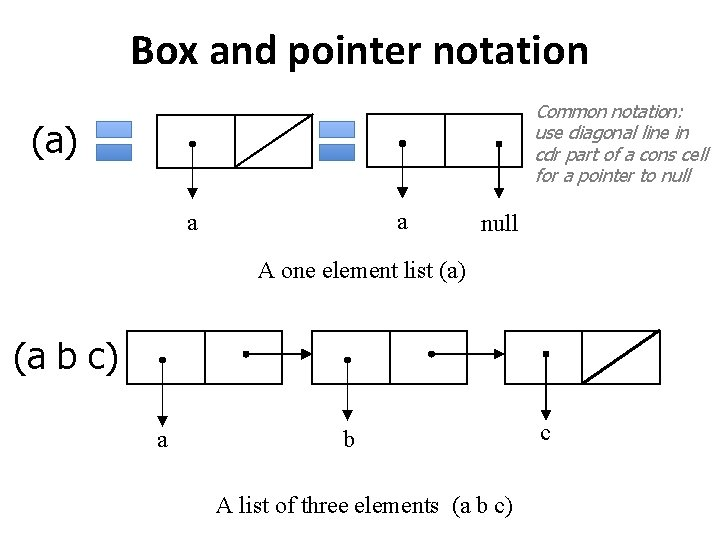Box and pointer notation Common notation: use diagonal line in cdr part of a cons cell for a pointer to null (a) a a null A one element list (a) (a b c) a b A list of three elements (a b c) cWhat sort of list is this? Z d a b Z is a list with three elements: (i) the atom a, (ii) a list of two elements, b & c and (iii) the atom d. c > (define Z (list ‘a (list ‘b ‘c) ‘d)) >Z (a (b c) d) > (car (cdr z)) ? ?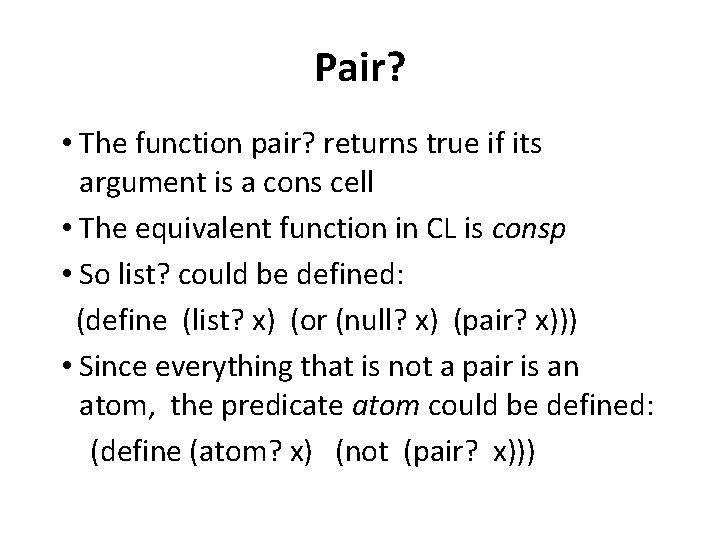Pair? • The function pair? returns true if its argument is a cons cell • The equivalent function in CL is consp • So list? could be defined: (define (list? x) (or (null? x) (pair? x))) • Since everything that is not a pair is an atom, the predicate atom could be defined: (define (atom? x) (not (pair? x)))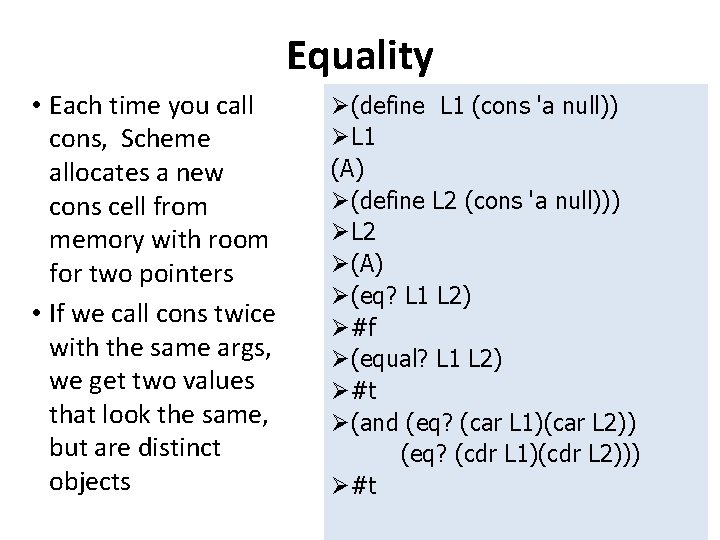Equality • Each time you call cons, Scheme allocates a new cons cell from memory with room for two pointers • If we call cons twice with the same args, we get two values that look the same, but are distinct objects Ø(define L 1 (cons 'a null)) ØL 1 (A) Ø(define L 2 (cons 'a null))) ØL 2 Ø(A) Ø(eq? L 1 L 2) Ø#f Ø(equal? L 1 L 2) Ø#t Ø(and (eq? (car L 1)(car L 2)) (eq? (cdr L 1)(cdr L 2))) Ø#t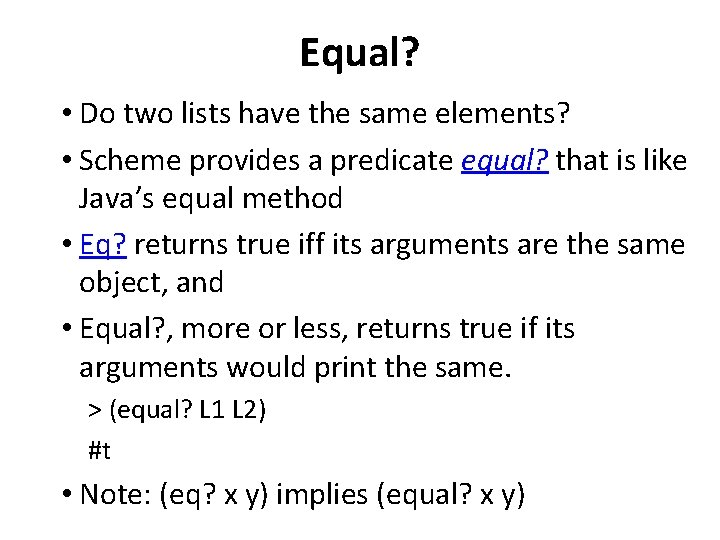Equal? • Do two lists have the same elements? • Scheme provides a predicate equal? that is like Java’s equal method • Eq? returns true iff its arguments are the same object, and • Equal? , more or less, returns true if its arguments would print the same. > (equal? L 1 L 2) #t • Note: (eq? x y) implies (equal? x y)Equal? (define (myequal? x y) ; this is ~ how equal? could be defined (cond ((number? x) (= x y)) ((not (pair? x)) (eq? x y)) ((not (pair? y)) #f) ((myequal (car x) (car y)) (myequal? (cdr x) (cdr y))) (#t #f)))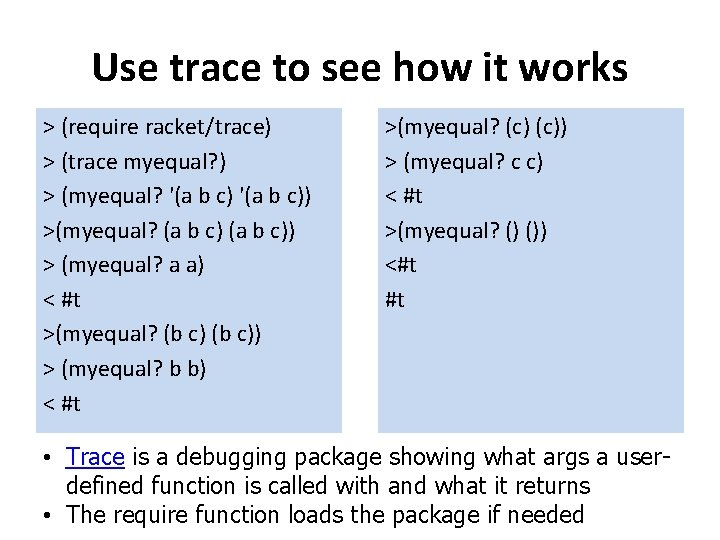Use trace to see how it works > (require racket/trace) > (trace myequal? ) > (myequal? '(a b c)) >(myequal? (a b c)) > (myequal? a a) < #t >(myequal? (b c)) > (myequal? b b) < #t >(myequal? (c)) > (myequal? c c) < #t >(myequal? () ()) <#t #t • Trace is a debugging package showing what args a userdefined function is called with and what it returns • The require function loads the package if neededDoes Lisp have pointers? • A secret to understanding Lisp is to realize that variables have values in the same way that lists have elements • As pairs have pointers to their elements, variables have pointers to their values • Scheme maintains a data structure representing the mapping of variables to their current values.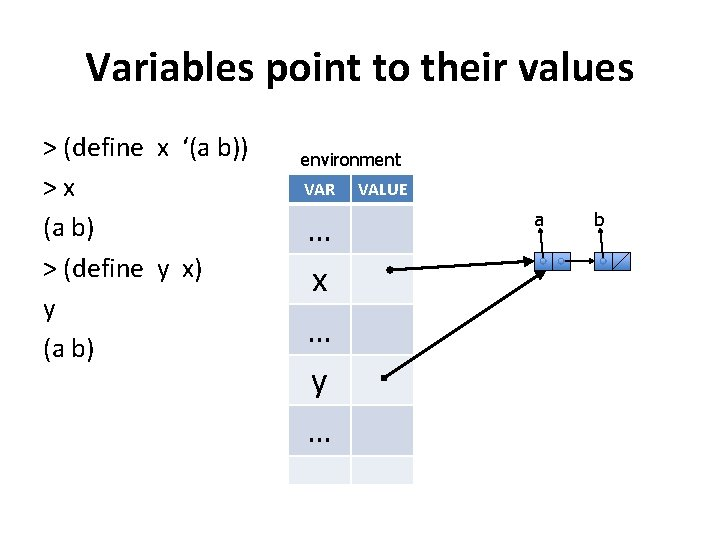Variables point to their values > (define x ‘(a b)) >x (a b) > (define y x) y (a b) environment VAR … x … y … VALUE a bVariables point to their values > (define x ‘(a b)) >x (a b) > (define y x) y (a b) > (set! y ‘(1 2) >y (1 2) environment VAR … x … y … VALUE a 1 b 2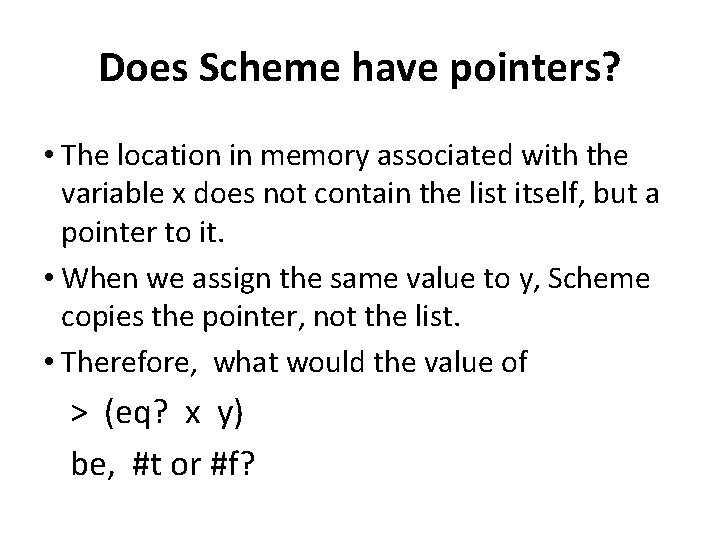Does Scheme have pointers? • The location in memory associated with the variable x does not contain the list itself, but a pointer to it. • When we assign the same value to y, Scheme copies the pointer, not the list. • Therefore, what would the value of > (eq? x y) be, #t or #f?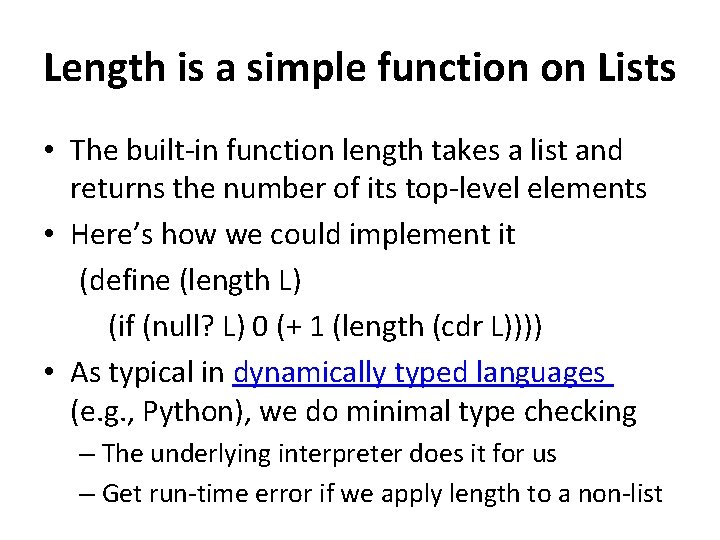Length is a simple function on Lists • The built-in function length takes a list and returns the number of its top-level elements • Here’s how we could implement it (define (length L) (if (null? L) 0 (+ 1 (length (cdr L)))) • As typical in dynamically typed languages (e. g. , Python), we do minimal type checking – The underlying interpreter does it for us – Get run-time error if we apply length to a non-listBuilding Lists • list-copy takes a list and returns a copy of it • The new list has the same elements, but contained in new pairs > (set! x ‘(a b c)) (a b c) > (set! y (list-copy x)) (a b c) • Spend a few minutes to draw a box diagram of x and y to show where the pointers point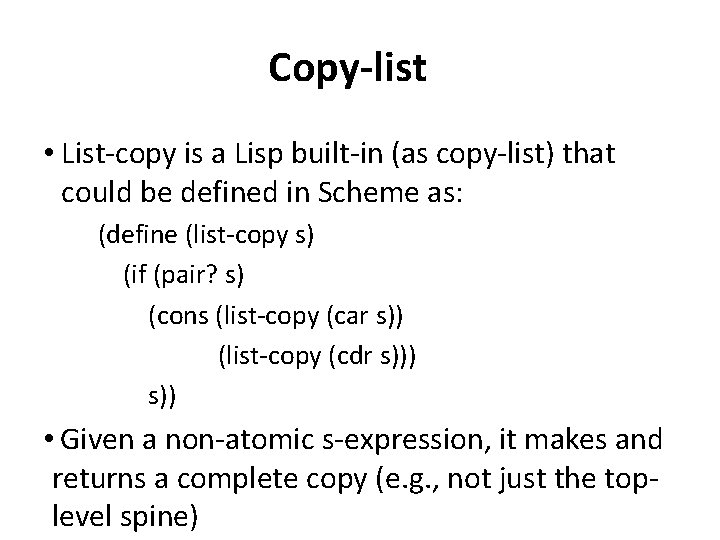Copy-list • List-copy is a Lisp built-in (as copy-list) that could be defined in Scheme as: (define (list-copy s) (if (pair? s) (cons (list-copy (car s)) (list-copy (cdr s))) s)) • Given a non-atomic s-expression, it makes and returns a complete copy (e. g. , not just the toplevel spine)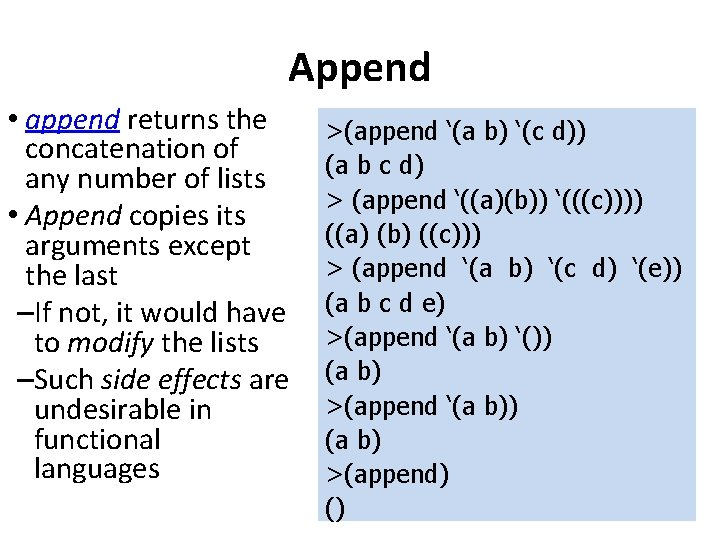Append • append returns the concatenation of any number of lists • Append copies its arguments except the last –If not, it would have to modify the lists –Such side effects are undesirable in functional languages >(append ‘(a b) ‘(c d)) (a b c d) > (append ‘((a)(b)) ‘(((c)))) ((a) (b) ((c))) > (append ‘(a b) ‘(c d) ‘(e)) (a b c d e) >(append ‘(a b) ‘()) (a b) >(append ‘(a b)) (a b) >(append) ()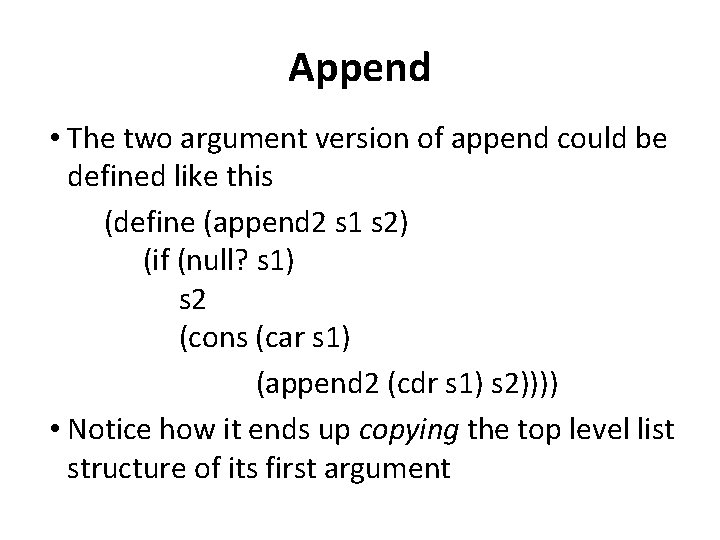Append • The two argument version of append could be defined like this (define (append 2 s 1 s 2) (if (null? s 1) s 2 (cons (car s 1) (append 2 (cdr s 1) s 2)))) • Notice how it ends up copying the top level list structure of its first argumentVisualizing Append > (load "append 2. ss") > (define L 1 '(1 2)) > (define L 2 '(a b)) > (define L 3 (append 2 L 1 L 2)) > L 3 (1 2 a b) > L 1 (1 2) > L 2 (a b) > (require racket/trace) > (trace append 2) > (append 2 L 1 L 2) >(append 2 (1 2) (a b)) > (append 2 (2) (a b)) > >(append 2 () (a b)) < <(a b) < (2 a b) <(1 2 a b) Append does not modify its arguments. It makes copies of all of the lists save the last.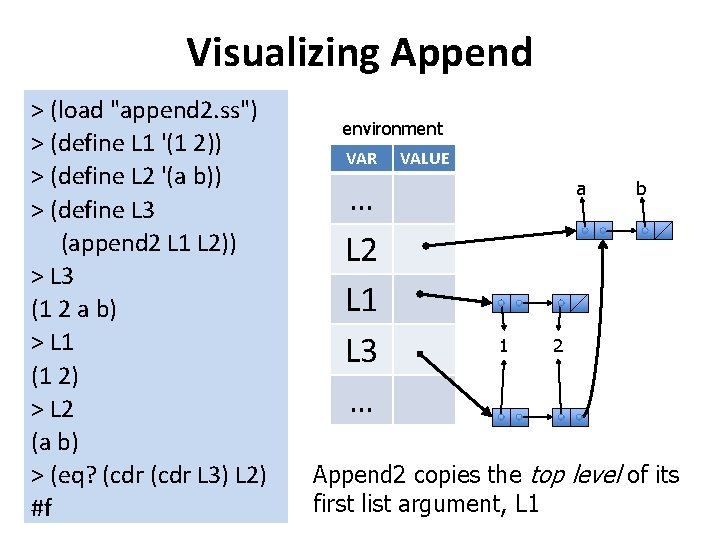Visualizing Append > (load "append 2. ss") > (define L 1 '(1 2)) > (define L 2 '(a b)) > (define L 3 (append 2 L 1 L 2)) > L 3 (1 2 a b) > L 1 (1 2) > L 2 (a b) > (eq? (cdr L 3) L 2) #f environment VAR … L 2 L 1 L 3 … VALUE a 1 b 2 Append 2 copies the top level of its first list argument, L 1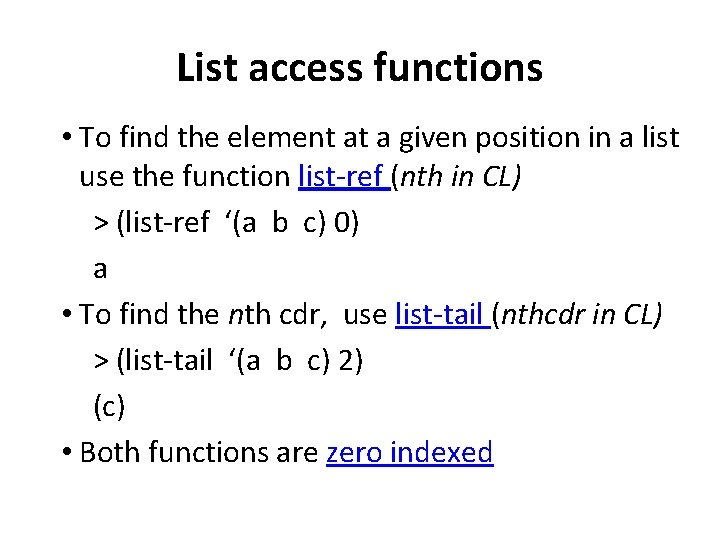List access functions • To find the element at a given position in a list use the function list-ref (nth in CL) > (list-ref ‘(a b c) 0) a • To find the nth cdr, use list-tail (nthcdr in CL) > (list-tail ‘(a b c) 2) (c) • Both functions are zero indexedList-ref and list-tail > (define L '(a b c d)) > (list-ref L 2) c > (list-ref L 0) a > (list-ref L -1) list-ref: expects type <non-negative exact integer> as 2 nd arg, given: -1; other arguments were: (a b c d) > (list-ref L 4) list-ref: index 4 too large for list: (a b c d) > (list-tail L 0) (a b c d) > (list-tail L 2) (c d) > (list-tail L 4) () > (list-tail L 5) list-tail: index 5 too large for list: (a b c d)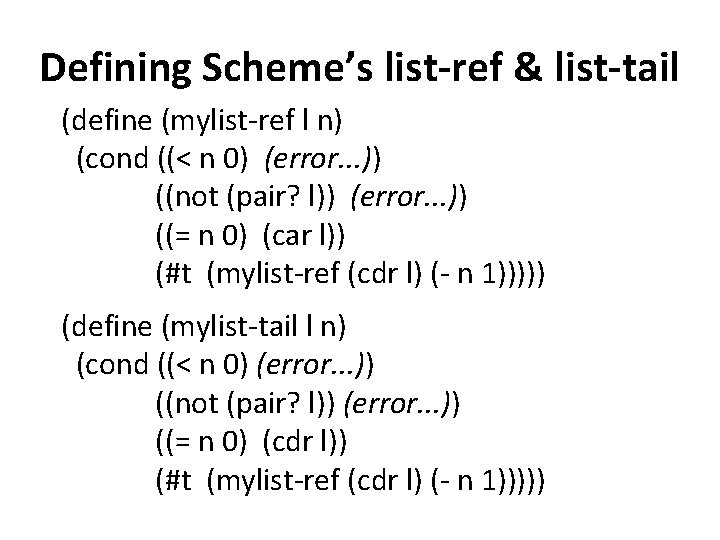Defining Scheme’s list-ref & list-tail (define (mylist-ref l n) (cond ((< n 0) (error. . . )) ((not (pair? l)) (error. . . )) ((= n 0) (car l)) (#t (mylist-ref (cdr l) (- n 1))))) (define (mylist-tail l n) (cond ((< n 0) (error. . . )) ((not (pair? l)) (error. . . )) ((= n 0) (cdr l)) (#t (mylist-ref (cdr l) (- n 1)))))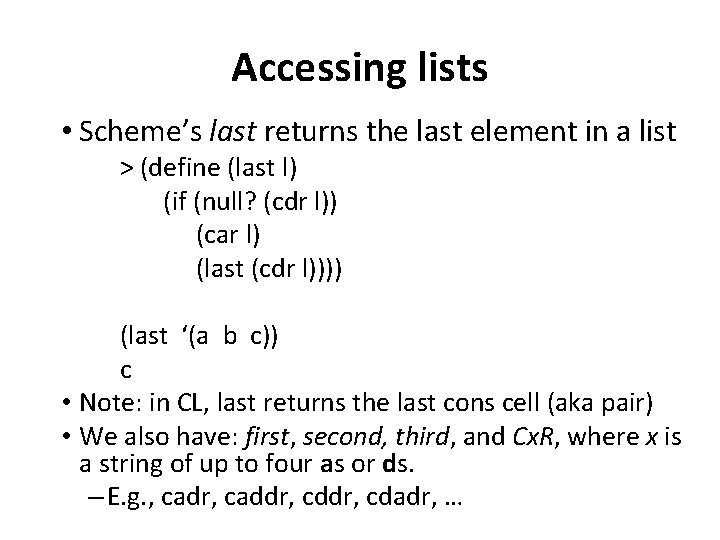Accessing lists • Scheme’s last returns the last element in a list > (define (last l) (if (null? (cdr l)) (car l) (last (cdr l)))) (last ‘(a b c)) c • Note: in CL, last returns the last cons cell (aka pair) • We also have: first, second, third, and Cx. R, where x is a string of up to four as or ds. – E. g. , cadr, caddr, cdadr, …Member • Member returns true, but instead of simply returning t, its returns the part of the list beginning with the object it was looking for. > (member ‘b ‘(a b c)) (b c) • member compares objects using equal? • There are versions that use eq? and eqv? And that take an arbitrary functionDefining member (define (member X L) (cond ((null? L) #f) ((equal? X (car L)) L) (#t (member X (cdr L)))))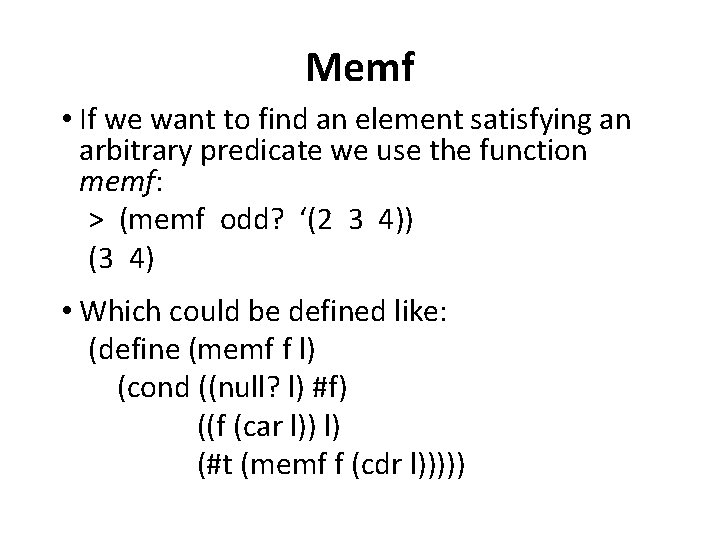Memf • If we want to find an element satisfying an arbitrary predicate we use the function memf: > (memf odd? ‘(2 3 4)) (3 4) • Which could be defined like: (define (memf f l) (cond ((null? l) #f) ((f (car l)) l) (#t (memf f (cdr l)))))Dotted pairs and lists • Lists built by calling list are known as proper lists; they always end with a pointer to null A proper list is either the empty list, or a pair whose cdr is a proper list • Pairs aren’t just for building lists, if you need a structure with two fields, you can use a pair • Use car to get the 1 st field and cdr for the 2 nd > (define the_pair (cons ‘a ‘b)) (a. b) • Because this pair is not a proper list, it’s displayed in dot notation In dot notation the car and cdr of each pair are shown separated by a period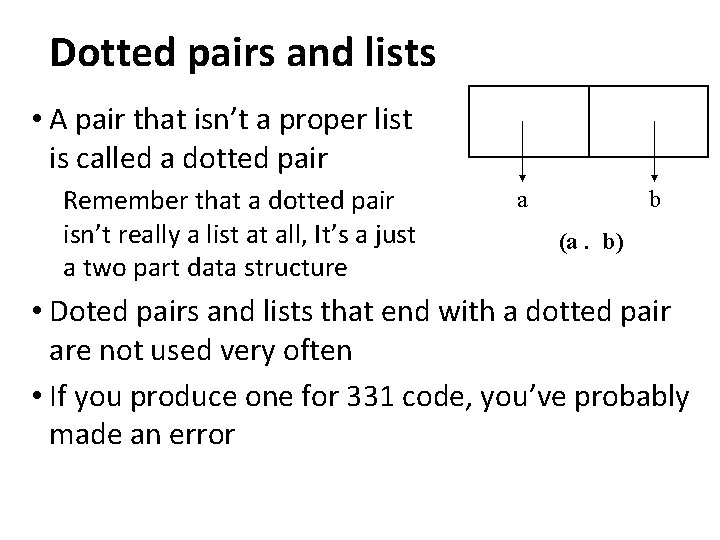Dotted pairs and lists • A pair that isn’t a proper list is called a dotted pair Remember that a dotted pair isn’t really a list at all, It’s a just a two part data structure a b (a. b) • Doted pairs and lists that end with a dotted pair are not used very often • If you produce one for 331 code, you’ve probably made an errorConclusion • Simple linked lists were the only data structure in early Lisps – From them you can build most other data structures though efficiency may be low • Its still the most used data atructure in Lisp and Scheme – Simple, elegant, less is more • Recursion is the natural way to process lists